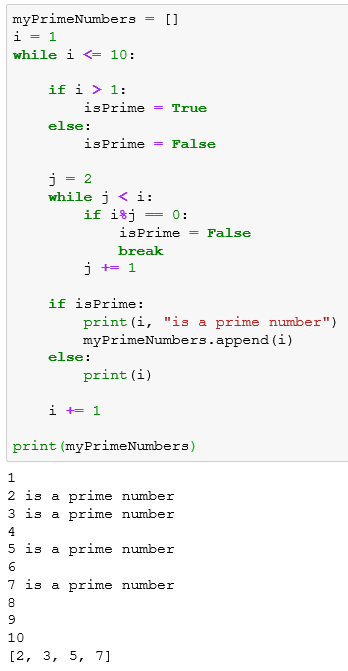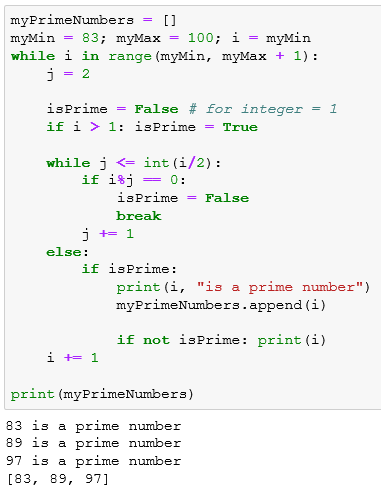﻿ How to Find Prime Numbers in Python using While LoopPython Programming and Python Code Tutorials Python Programming and Python Code Tutorials for Programmers

# How to Find Prime Numbers in Python using While Loop

In this Python tutorial, I want to share source codes of a simple Python program which identifies whether a number is a prime number or a composite number. Python programmers can execute the prime number checker program for numbers smaller than an integer value, or for a given range of integer numbers. The WHILE Loops and conditional IF statements will help us to build our sample Python program.

## Prime Numbers and Composite Numbers

If a positive integer number which is greater than 1 cannot be divided by any integer except 1 and itself, this integer is called as a prime number. If the positive integer has at least an other divisor rather than 1 and itself, this number is called as a composite number

In this case number 1 is neither a prime number nor a composite number

For example, 1 is not a prime number since it has only 1 divisor.
2 is a prime number because it can only be divided into 1 and 2.
3 is a prime number because its divisor numbers are 1 and 3.
But 4 is a composite number. In addition to 1 and the number itself, 4 can also be divided by 2. This makes 4 a composite number preventing it to be a prime number.

## Python Code to Find Prime Numbers

Below is the execution output of the above Python code block on a Jupyter notebook## Python Prime Number Checker Program

Of course the first version of our sample Python code which identifies the prime numbers and adds them into a list object, can be optimized further. In following Python code, I added the option to find prime numbers for a given range. So Python developers don't have to search for prime numbers starting from integer 1 everytime. Additionally, while searching for divisors of the number, we can limit our searches in inner WHILE loop until we reach to half of the original integer value.

Here is the modified Python source code for the prime number checker program

And below screenshot from the Jupyter notebook shows the output of the Python prime number checker program for the range of integers between 83 and 100I hope the above two sample Python code blocks helps developers to understand how they can find prime numbers in a given range of integer values and how they can use WHILE loop with list objects and IF condiitonal statements.

Python Tutorials